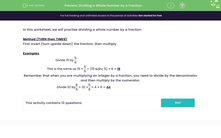# Divide a Whole Number by a Fraction

In this worksheet, students will practise dividing whole numbers by fractions.Key stage:  KS 3

Curriculum topic:   Number

Curriculum subtopic:   Use Four Operations for All Numbers

Difficulty level:#### Worksheet Overview

In this activity, we will practise dividing a whole number by a fraction.

The method we're going to use is KFCNo, not the takeaway chicken - something much better!

KFC stands for Keep Flip Change

First, we keep the first number or fraction unchanged.

Next, we flip the second fraction upside down.

Finally, we change the sign between the two fractions or numbers from ÷ to x.

If we are dividing whole numbers by fractions, we just keep the whole number unchanged, just as you would if it had been a fraction.

Examples

15 ÷ 5/6

Keep the whole number as it is: 15

Flip the fraction you're dividing by: 6/5

Change the sign to x

Now we have 15 x 6/5

The rule for multiplying a whole number by a fraction is divide the big number by the bottom number and multiply by the top number.

15 x 6/5

15 ÷ 5 = 3

3 x 6 = 18

So 15 ÷ 5/6 = 18

Let's look at another one.

32 ÷ 8/11

Keep 32 unchanged: 32

Flip the fraction you're dividing by: 11/8

Change the sign to x

32 x 11/8

Divide the big number by the bottom number: 32 ÷ 8 = 4

Multiply by the top number: 4 x 11 = 44

So, 32 ÷ 8/11 = 44

Shall we have a go at some questions?

### What is EdPlace?

We're your National Curriculum aligned online education content provider helping each child succeed in English, maths and science from year 1 to GCSE. With an EdPlace account you’ll be able to track and measure progress, helping each child achieve their best. We build confidence and attainment by personalising each child’s learning at a level that suits them.

Get started